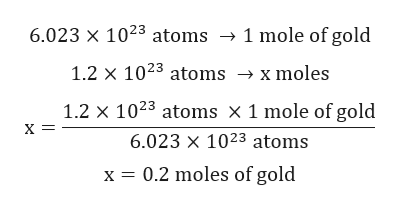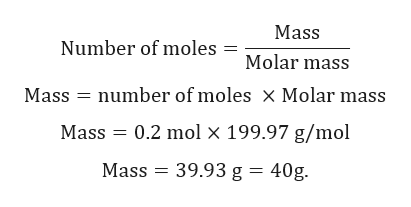1.2x10^23 gold atoms calculate into mass, in grams

Question

1.2x10^23 gold atoms calculate into mass, in grams

Step 1

Given:

Number of gold atoms = 1.2 x 1023 atoms.

Molar mass of gold (Au) = 199.97 g/mol.

Step 2

Calculation for number of moles of gold:help_outlineImage Transcriptionclose6.023 x 1023 atoms -» 1 mole of gold 1.2 x 1023 atoms x moles 1.2 x 1023 atoms x 1 mole of gold х- 6.023 x 1023 atoms x 0.2 moles of gold fullscreen
Step 3

Calculation fo...help_outlineImage TranscriptioncloseMass Number of moles = Molar mass number of moles x Molar mass Mass Mass 0.2 mol x 199.97 g/mol Mass 39.93 g = 40g fullscreen

Want to see the full answer?

See Solution

Want to see this answer and more?

Our solutions are written by experts, many with advanced degrees, and available 24/7

See Solution
Tagged in

General Chemistry